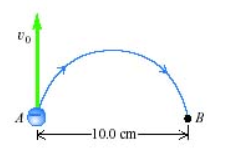# Problem: An electron at point A in the figure has a speed of 1.49 × 106 m/s.(a) Find the direction of the magnetic field that will cause the electron to follow the semicircular path from A to B.(b) Find the magnitude of the magnetic field that will cause the electron to follow the semicircular path from A to B.(c) Find the time required for the electron to move from A to B.(d) Find the direction of the magnetic field, that would be needed if the particle were a proton instead of an electron?(e) Find the magnitude of the magnetic field, that would be needed if the particle were a proton instead of an electron?

###### FREE Expert Solution

(a) Lorentz force:

$\overline{)\begin{array}{rcl}{\stackrel{\mathbf{⇀}}{\mathbf{F}}}_{\mathbf{B}}& {\mathbf{=}}& \mathbf{q}\stackrel{\mathbf{⇀}}{\mathbf{v}}\stackrel{\mathbf{⇀}}{\mathbf{B}}\mathbf{s}\mathbf{i}\mathbf{n}\mathbf{\theta }\end{array}}$

92% (129 ratings)###### Problem DetailsAn electron at point A in the figure has a speed of 1.49 × 106 m/s.

(a) Find the direction of the magnetic field that will cause the electron to follow the semicircular path from A to B.

(b) Find the magnitude of the magnetic field that will cause the electron to follow the semicircular path from A to B.

(c) Find the time required for the electron to move from A to B.

(d) Find the direction of the magnetic field, that would be needed if the particle were a proton instead of an electron?

(e) Find the magnitude of the magnetic field, that would be needed if the particle were a proton instead of an electron?